This tutorial has two parts. In the first part you’ll learn how to plot functions in R. In the second part you’ll learn how we can use R to study the Binomial random variable.

## Plotting Curves in R

You’ve already seen a number of examples of the plot function in R. So far we’ve mainly plotted points but we can actually use the same command to plot functions. The basic idea is to set up the x and y coordinates of some points that are close enough together that it looks like we’ve plotted a smooth curve. Everything on a computer is actually discrete: your eye is simply fooled into thinking that things look continuous. All curves are actually many tiny line segments!

### Simple Example

Let’s start by plotting a few points on the curve $$f(x) = x^2$$

x <- seq(from = -1, to = 1, by = 0.5)
y <- x^2
plot(x, y)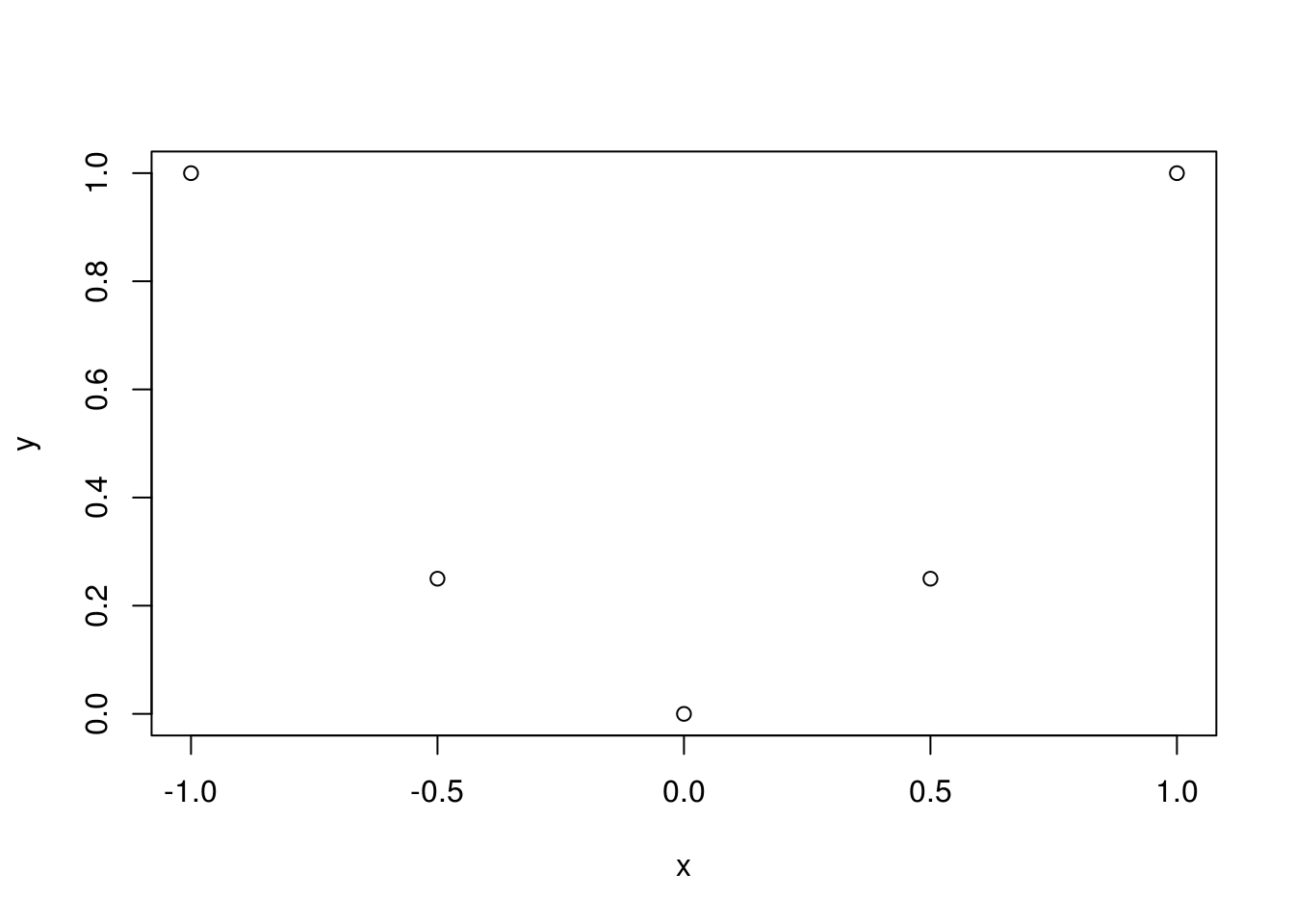Those points aren’t “dense” enough to approximate the whole curve. Let’s try making a finer plot:

x <- seq(from = -1, to = 1, by = 0.1)
y <- x^2
plot(x, y)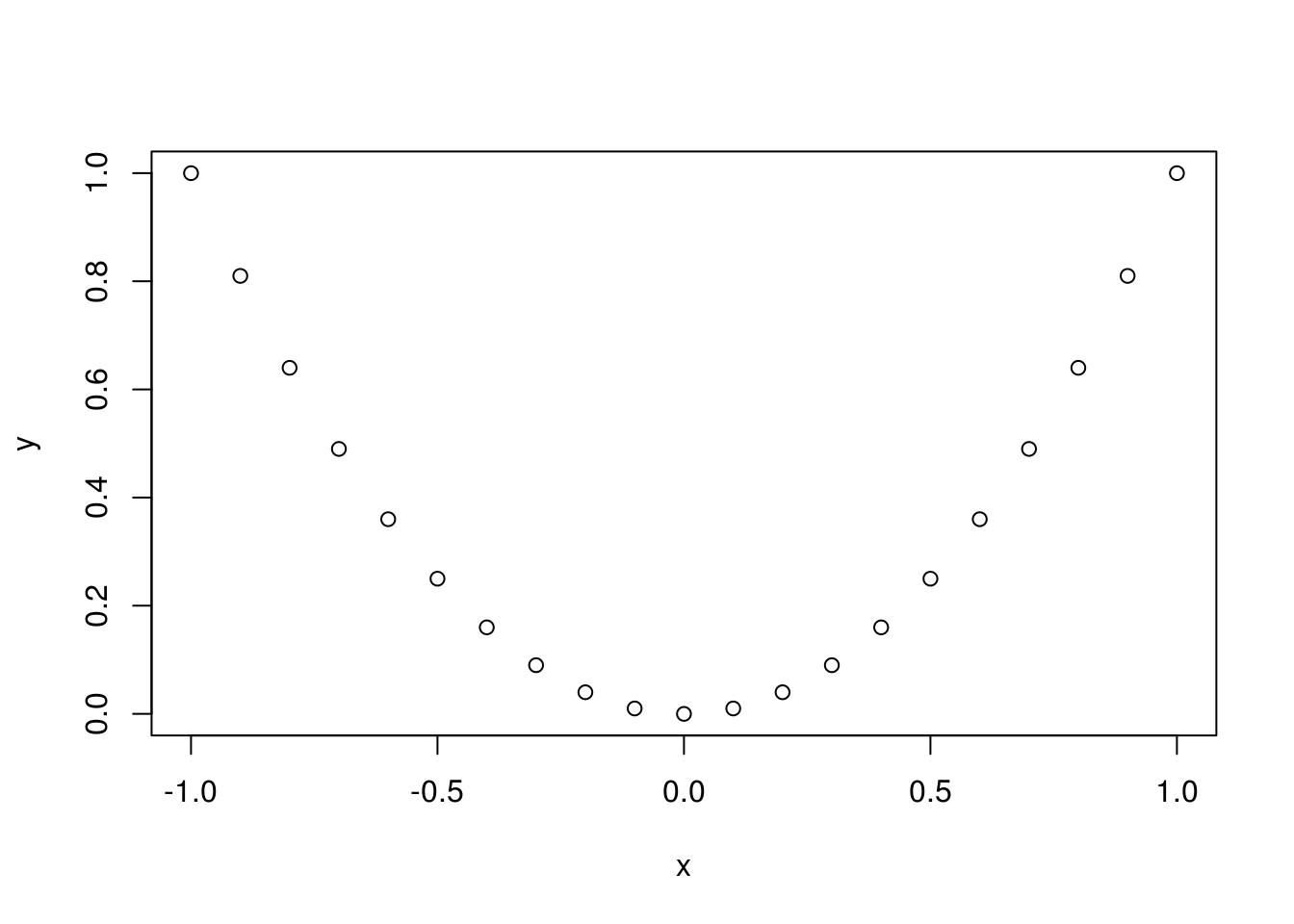This looks better, but how about even finer?

x <- seq(from = -1, to = 1, by = 0.01)
y <- x^2
plot(x, y)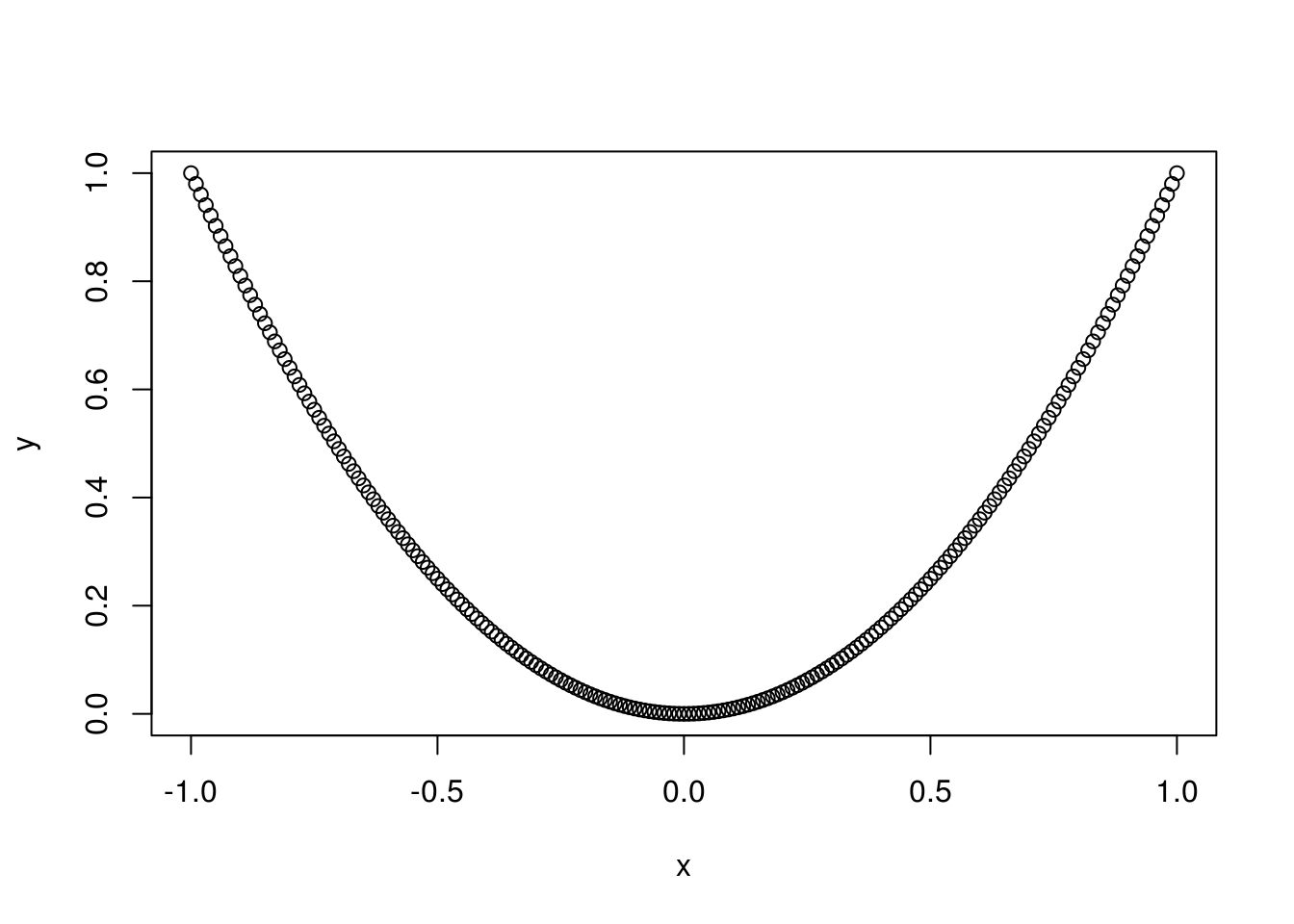This is more like what we’re after, but we’d rather have a smooth curve rather than all those little “dots.” We can do this as follows:

plot(x, y, type = "l")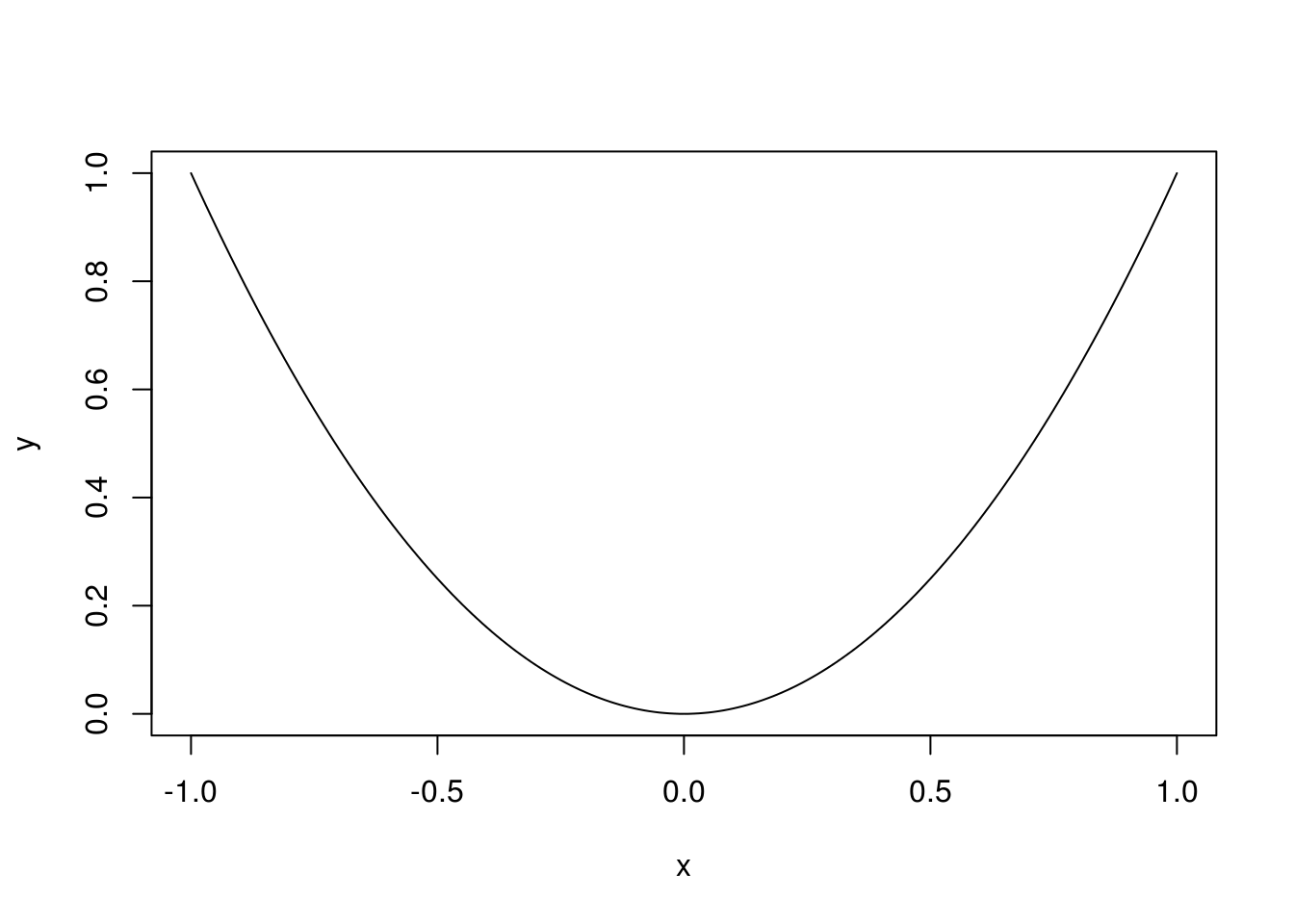### Exercise #1

Plot $$f(x) = x^3$$ on $$[-2,2]$$.

x <- seq(from = -2, to = 2, by = 0.01)
y <- x^3
plot(x, y, type = 'l')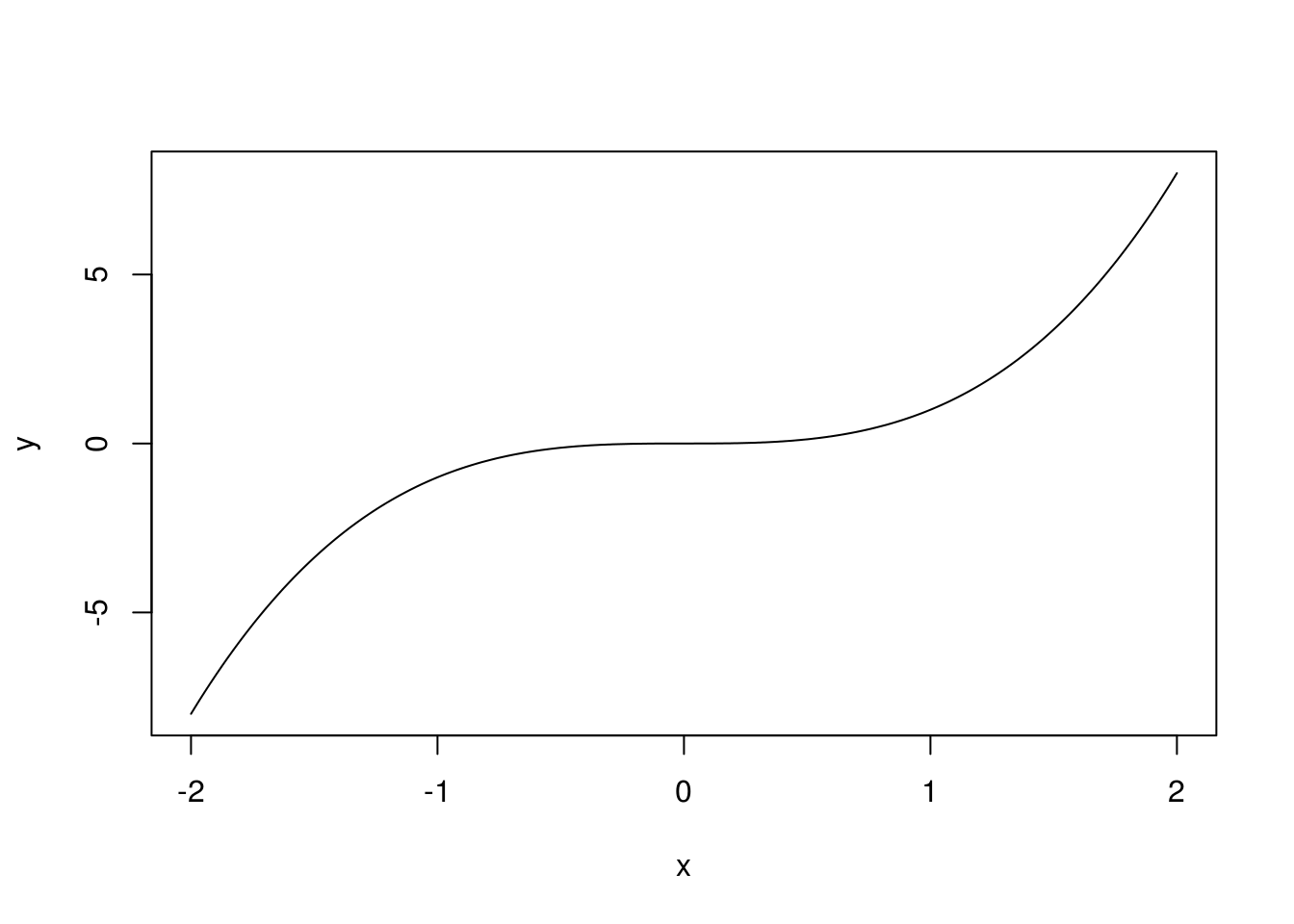### Exercise #2

Plot $$f(x) = \log(x)$$ on $$[0.5, 1.5]$$.

x <- seq(from = 0.5, to = 1.5, by = 0.01)
y <- log(x)
plot(x, y, type = 'l')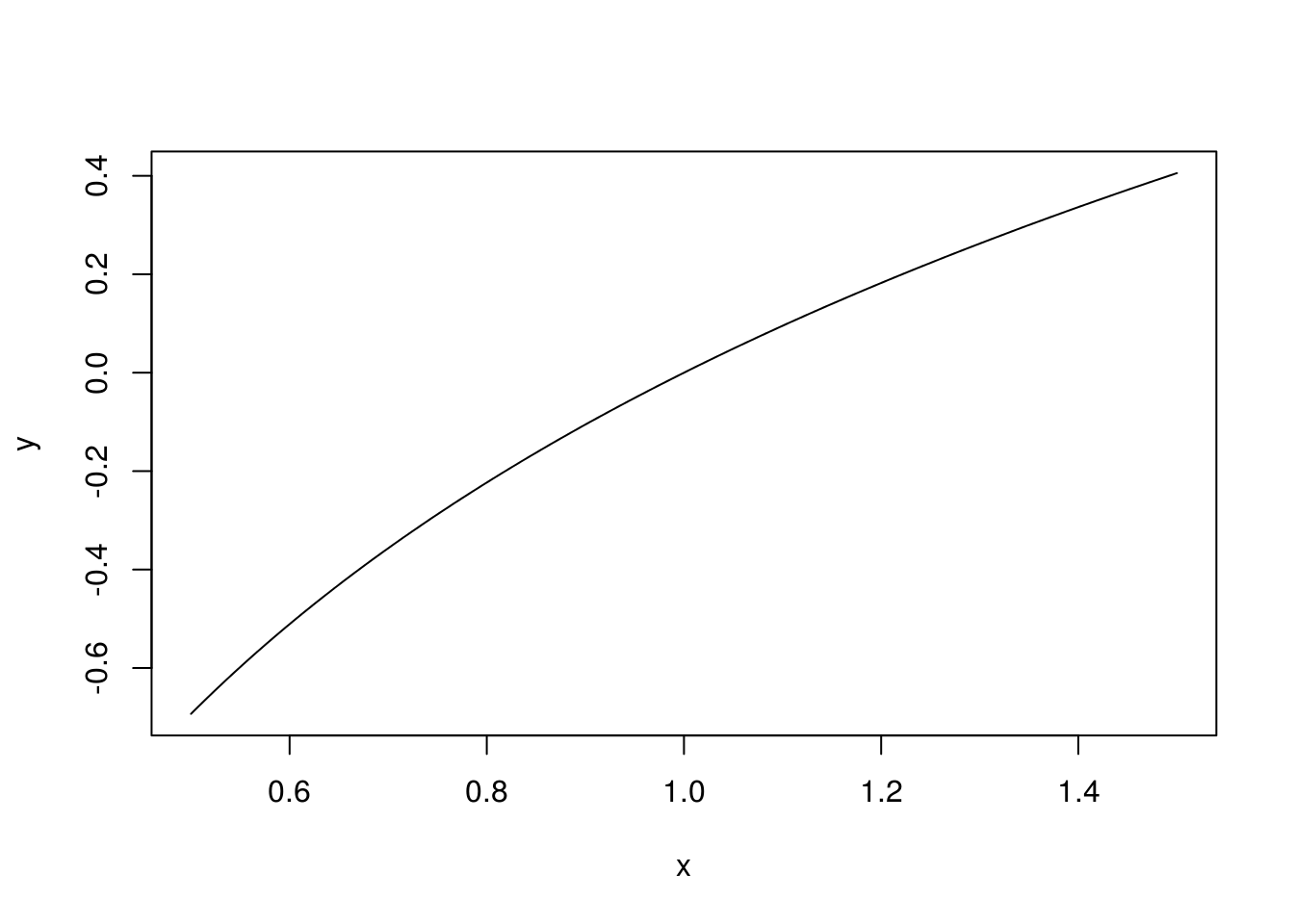### Exercise #3

Plot $$f(x) = \sin(x)$$ on $$[0, 6\pi]$$.

x <- seq(from = 0, to = 6 * pi, by = 0.01)
y <- sin(x)
plot(x, y, type = 'l')